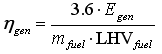# HOMER Grid 1.1

 Navigation: Glossary Generator Average Electrical EfficiencyType: Output Variable Units: % Symbol: ηgen

The generator average electrical efficiency is the average of the generator's electrical efficiency over a year, defined as the electrical energy out divided by fuel energy in. HOMER uses the following equation to calculate the average electrical efficiency:where: Egen = the generator's total annual electrical production [kWh/yr] mfuel = the generator's total annual fuel consumption [kg/yr] LHVfuel = the lower heating value of the fuel [MJ/kg]

We use 3.6 in the equation above because 1 kWh = 3.6 MJ.

See also:

Generator Average Total Efficiency

The Homer Support site has a searchable knowledgebase and additional support options. HOMER Online contains the latest information on model updates, as well as sample files, resource data, and contact information. ©2017 HOMER Energy, LLC